## Introduction

Most stable carbon allotropes, including the prominent members such as graphite, diamond, carbon nanotube1 and graphene2, possess a motif of hexagonal rings due to the dominating sp2 and sp3 bonding character3. In contrast, the motif of pentagonal rings, although can appear in molecules such as fullerene4, is very rare in three-dimensional (3D) allotropes. This is partly due to the unfavourable bonding angles that may easily cause structural instability5, and also because the pentagonal motif does not readily fit into the 3D space group symmetry6. It seems unlikely to have a stable 3D carbon allotrope dominated by pentagonal carbon rings.

Meanwhile, the research on topological metals and semimetals (TMs) has been attracting tremendous interest7,8,9,10,11,12,13,14. In these materials, topologically protected quasiparticles emerge at protected band-crossing points around Fermi Level, giving rise to condensed matter realizations of many peculiar fermions with a variety of distinct electronic properties. For example, in Dirac and Weyl semimetals, the quasiparticles near the protected linear band-crossing points are direct analogues of the Dirac and Weyl fermions in relativistic quantum field theory, providing unique opportunities to study interesting high-energy physics phenomena such as chiral anomaly and gravitational anomaly in a table-top experiment15,16,17,18. The emergent topological fermions can be classified by the degeneracy of the band-crossing point, which dictates the number of internal degrees of freedom of the fermion. Dirac and Weyl fermions are associated with crossing points with fourfold and twofold degeneracies19,20. Importantly, the breaking of Lorentz symmetry at lattice scale makes it possible to have TMs that can host new types of fermions with different degeneracies beyond the Dirac and Weyl fermions21, 22, 23. These new fermions are now actively searched for, and if realized, they are believed to host a wealth of exotic physical properties unprecedented in high-energy physics.

The search for TMs has been focused on compounds involving heavy elements7,24,25, hoping that the strong spin–orbit coupling could help to achieve a nontrivial band structure. On the other hand, light elements such as carbon may offer a distinct alternative. For example, graphene is well-known with two-dimensional (2D) Dirac fermions26. With negligible spin–orbit coupling, the real spin in carbon materials can be considered as a dumb degree of freedom27. Then the fundamental time reversal operation (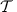) here satisfies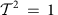, contrasting with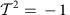for the spinful case. Thus in terms of topological classifications, the nontrivial phases in carbon would be fundamentally distinct from those in spin–orbit-coupled systems. Indeed, previous studies have identified a few TM carbon allotropes with Weyl points28,29, nodal loops27,29,30,31,32 and even Weyl surfaces28. Motivated by these observations, one may naturally wonder: is it possible to also realize new types of topological fermions in carbon allotropes?

In this work, based on first-principles calculations, we propose a new 3D carbon allotrope, named as 3D Pentagon Carbon (3D-C5), which is entirely composed of pentagonal carbon rings. With nicely interlaced structures, the structure shows good stability comparable to other metastable carbon allotropes. We characterize its phononic, mechanical and electronic properties. At the equilibrium state, Pentagon Carbon is a narrow-gap semiconductor. A semiconductor–metal quantum phase transition can be driven by an applied strain. Remarkably, at the critical transition point, three bands cross at a single Fermi point in the energy-momentum space, making the emergent low-energy fermions a novel type of isospin-1 triplet fermions. Beyond the transition, the single Fermi point splits into two triply degenerate band-crossing points along the fourfold screw-rotational axis. The material is turned into a novel TM possessing another type of triply degenerate fermions. When further breaking the screw-rotational symmetry, the triply degenerate fermion points will transform into two inter-connected Hopf-link Weyl loops protected by the remaining mirror symmetry. We show that, for each case, the Landau level (LL) spectrum exhibits distinct features tied to the topological fermions, suggesting pronounced anomalies in magneto-transport experiment. In addition, we discuss a possible reaction pathway for the experimental synthesis of Pentagon Carbon.

## Results

### Lattice structure and stability

The structure of 3D Pentagon Carbon is shown in Fig. 1a , which can be viewed as formed by interlinking two orthogonal arrays of pentagon-ring nanoribbons and stacking them along the z-direction (Fig. 1b). After linking, the two nanoribbons share one (green-colored) atom. Hence in the Pentagon Carbon lattice, there are two kinds of carbon atoms, that is, the sp2-hybridized (red- and blue-colored) atoms forming the backbones of the pentagon-ring nanoribbons and the sp3-hybridized (green-colored) atoms forming the linkage between ribbons.Figure 1: Crystal structure and Brillouin zone of 3D Pentagon Carbon.

The structure has the nonsymmorphic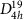space group symmetry (No. 141, I41/amd). Here an important symmetry element is the screw rotation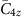, which is a fourfold rotation along z followed by a factional lattice translation of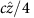, where c is the lattice parameter in the z direction. The detailed structural properties are presented in Table 1 and are compared with several other (meta-) stable 3D carbon allotropes. One observes that the cohesive energy of Pentagon Carbon, although slightly higher than graphite and diamond, is less than T6 (ref. 33) and Bcc-C8 (ref. 34) and is comparable with Carbon Kagome Lattice (CKL)35,36 structure. Due to its porous structure, the mass density of Pentagon Carbon is low (2.18 g cm−3) comparable with that of graphite. This is also correlated with its relatively low bulk modulus 257 GPa compared with other allotropes (except for graphite), suggesting that external strain can be more easily applied. The phonon spectrum of Pentagon Carbon is also investigated, which shows no soft mode, confirming its dynamical stability (more analysis of lattice structure and stability can be found in Supplementary Fig. 1 and Note 1).

### Electronic structure and quantum phase transition

Remarkably, Pentagon Carbon is also a material hosting a series of novel topological fermions beyond the usual Dirac and Weyl fermions. The band structure in Fig. 2a shows that, in equilibrium state, it is a narrow-gap semiconductor with a small energy gap 21.4 meV. The electronic structure shows strong anisotropy between in-plane (xy) and out-of-plane (z) directions in accordance with the crystal structural anisotropy. Focusing on the Γ point, there are two valence bands (heavy-hole and light-hole) and a single conduction band at low energy, with the conduction band and the light-hole valence band almost crossing linearly. Note that the degeneracy of the two valence bands at Γ is dictated by symmetry: the two states belong to a 2D irreducible representation Eg of the D4h group at Γ. In comparison, the conduction band belongs to the one-dimensional (1D) A1u representation. Another salient feature is that the two valence bands are degenerate along the Γ–Z path (kz-axis). This is because that there is remaining C4v little group symmetry along this screw axis, such that the 2D representation does not split according to the compatibility relation. From the analysis of wave-function and projected density of states (PDOS), we find that the low-energy states are mainly from the π orbitals of the sp2 atoms (Fig. 2f, Supplementary Fig. 2).

Since the semiconducting gap is small, a slight perturbation may close the gap and drive a semiconductor-to-metal phase transition (a tight-binding model analysis is given in Supplementary Figs 3,4 and Note 2). In Fig. 2b–d, we show band structures for three applied uniaxial strains ɛ=1, −0.1 and −1% along the z direction. One observes that indeed a semiconductor–metal phase transition is induced. A tensile strain drives the system to a semiconductor with wider gap, whereas a compressive strain drives the system towards a metal. Of particular interest is the change of band ordering at the Γ point. The A1u singlet state and the Eg doublet switch their positions in energy across the transition. Due to their different symmetry character, A1u and Eg states cannot hybridize in the transition. Hence at the critical strain, we must have the three bands crossing at a single point, where the conduction and the light-hole bands have linear dispersion in the kx–ky plane; and all three bands have quadratic dispersion along the screw axis. The 3D band structures in Fig. 2i,j clearly show the anisotropic feature around the triplet point.

Passing the critical point, the band ordering is inverted at Γ. However, the corresponding states at Z on the Brillouin zone boundary still have the original ordering. This band topology means that the two bands must cross each other between Γ and Z. Further, because the two bands still belong to different representations along the screw axis, they must cross in a linear manner with two triply degenerate band-crossing points (one on each side of Γ), as confirmed in Fig. 2d. Therefore, the metallic phase represents a novel TM phase with a pair of triply degenerate band-crossing points near the Fermi level. In such sense, the quantum phase transition may also be regarded as a topological phase transition from a trivial semiconductor phase to a TM phase.

We can define a Z2 invariant to characterize this TM phase and the phase transition. Let Δ and Δz be the values of the energy difference between A band and E band at Γ point and at Z point, respectively. Then a Z2 invariant can be defined as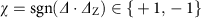.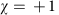corresponds to the trivial case where a band-crossing point does not need to occur, while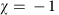is the topologically nontrivial case where a triply degenerate crossing point must exist between Γ and Z. The topological phase transition from a trivial semiconductor phase to TM phase (as shown in Fig. 2) is thus characterized by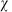changing from +1 to −1. The topological character is manifest in the sense that as long as the Z2 invariantis well defined (that is, symmetry is preserved) and does not change, the existence of the triply degenerate crossing point and the linear band dispersion along kz near the point are guaranteed and insensitive to system parameters and perturbations.

### Emergent fermions and k·p model

Nontrivial band-crossing points are fascinating objects because the fermionic quasiparticles around these points necessarily have a multi-component structure, distinct from the usual Schrodinger fermions. The essential physics of these emergent fermions are characterized by the low-energy effective k·p model expanded around the crossing point.

Around the Γ point, we can construct the k·p model based on the three basis states of A1u and Eg. Constrained by the D4h symmetry group and the time reversal symmetry for a spinless system (as spin–orbit coupling is negligible for carbon), one obtains the k·p model up to k-quadratic order as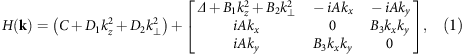where the coefficients A, B1, B2, C, D1, D2, and Δ can be determined by fitting the density functional theory (DFT) result. At transition point, Δ=0, the three bands cross at a single point, as illustrated in Fig. 3a. Then we can further simplify the model by keeping only the k-linear terms: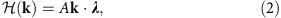where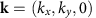, and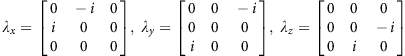are three of the eight Gell-Mann matrices corresponding to spin-1 (ref. 37). This describes an isospin-1 triplet fermion moving in the xy plane. The fermion is helical, with a well-defined helicity of ±1 and 0 corresponding to the eigenvalues of the helicity operator k·λ/k. The two branches with helicity ±1 are massless while the remaining one with helicity 0 has a flat dispersion, and these are constrained by the presence of an emergent ‘chiral symmetry’ operation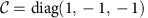with. The finite mass term for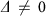breaks this symmetry, generating a mass for both ±1 branches and gapping the spectrum.

Passing the critical point, the isospin-1 triplet fermion point split into two triply degenerate points located at kz=τKc, as shown in Fig. 3b, where τ=± is an index labelling the two crossing points and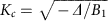(note that we must have Δ<0 and B1>0 to reproduce the band structure). The emergent triply degenerate fermions around each crossing point are described by the linearized model obtained from equation (1) as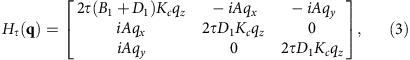where the wave vector q is measured from the crossing point. One notes that in the direction perpendicular to the screw axis, that is, in the qxqy plane, the dispersion is the same as that for the isospin-1 triplet fermion in equation (2). One finds that the triply degenerate fermions basically inherit the structure of the triplet fermions but acquire one more degree of freedom, that is, the motion along the z direction. In the meantime, they lose the well-defined helicity.

The two kinds of fermionic quasiparticles, the isospin-1 triplet fermions and the triply degenerate fermions, are new types of emergent fermions. Unlike the Dirac and Weyl fermions studied before, these new fermions do not have direct analogues in the relativistic quantum field theory. The isospin-1 triplet fermion point is not topologically protected: it marks the critical point in the quantum phase transition. In contrast, the two triply degenerate fermion points are protected by the nontrivial band topology and the fourfold screw axis. These features are schematically illustrated in Fig. 3a,b.

When further breaking the screw-rotational symmetry, the double degeneracy of the E band will be lifted; hence the triply degenerate fermion point will disappear. However, note that the original A and E bands have different eigenvalues under the mirror operations Mxz and Myz. Hence crossings between each split E band and A band in the mirror-invariant kxkz and kykz planes will be protected if the respective mirror symmetry remains. For example, by an additional uniaxial strain along x direction or along diagonal directions in the x–y plane, we find that, interestingly, the resulting band crossings form two orthogonal concatenated Weyl loops, which has the topology of a Hopf link. As shown in Fig. 3c, for an additional tensile strain along the x direction, one Weyl loop is centred at the Γ point lying in the kx–kz plane, while the other loop is in the ky–kz plane and crosses the first Brillouin zone boundary. Around each point on the loop, the energy dispersion along two transverse directions is of the Weyl-like linear type as shown in Fig. 3d. It needs to be emphasized that the band crossing forms the Weyl-loop fermion, not Weyl fermion, because the inversion symmetry is still preserved. It is particularly interesting that the two Weyl loops form an inter-connected Hopf-link pattern, and these two loops are protected by the Mxz and Myz mirror symmetries, respectively. It should also be noted that there is no symmetry to pin these Weyl loops at a constant energy, and, indeed, the energy varies along each loop.

### Landau level spectrum

Topological character of the band structure can manifest when subjecting to a magnetic field38,39,40. For a 3D system, the electron motion in the plane perpendicular to the field is quantized into LLs. The dispersion along the field connects the levels to form dispersive Landau bands. For example, at each Weyl point, there is a gapless chiral Landau band, such that an additional parallel electric field will drive charge creation or depletion depending on the chirality. Since Weyl points appear in pairs of opposite chirality, the process leads to charge pumping between the Weyl points. This non-conservation of chiral charge at a single Weyl point is the well-known chiral anomaly14,18,40. Experimentally, it manifests as a negative magnetoresistance, which has recently been demonstrated in Weyl semimetals15,16.

For Pentagon Carbon, considering a magnetic field along the screw axis such that the screw rotational symmetry is preserved. Focusing on the low-energy physics around the Γ point where the quantum phase transition occurs, we find that the LL spectrum exhibits drastic difference across the transition. As illustrated in Fig. 4a, one observes that in the semiconductor phase, the LL spectrum shows a spectral gap, typical for a semiconducting state. Remarkably, in the TM phase, there appear two chiral Landau bands crossing the spectral gap and crossing the location of the original triply degenerate fermion points (Fig. 4b). This is like that of a Weyl point; however, there is a marked difference: the chiral band here is in fact with a huge number of Landau bands nearly degenerate onto a single curve21. This behaviour is due to the small values of the in-plane quadratic terms such as B2 and B3. If one artificially increases these parameters, there will be a visible splitting between the almost degenerate Landau bands. Similar to chiral anomaly, a parallel E-field would pump charges from one triply degenerate point to the other. Due to the large number of the bands, it is expected to generate pronounced signals in magneto-transport experiment.

When further breaking the screw-rotational symmetry, the triply degenerate fermion points disappear, giving rise to inter-connected Hopf-link Weyl loops. In the LL spectrum, we see that the main effect of the symmetry breaking is to split the chiral Landau bands, as indicated in Fig. 4c. These interesting LL features reflect the evolution of the band-crossing points and will be useful for probing the quantum phase transition and the emergent topological fermions.

## Discussion

Our work discovers a new metastable 3D carbon allotrope—the Pentagon Carbon, which exhibits a beautiful lattice structure entirely composed of pentagonal rings—a highly rare lattice motif for 3D crystals. Originating from this unique structure, there appear interesting quantum phase transitions and the associated novel emergent fermions. Remarkably, we reveal a fascinating transformation from isospin-1 triplet fermion point to triply degenerate fermion point and finally to inter-connected Hopf-link Weyl loops.

Nontrivial band-crossing point is also predicted in a few recent works. Particularly, Bradlyn et al.21 classified all possible degeneracy points at high-symmetry k-points for the 230 space groups in spin–orbit-coupled systems, and triple-degenerate points have also been found in a few materials with strong spin–orbit coupling22,41,42. The triply degenerate points here are distinct from those previous examples in the following aspects: first, due to the negligible SOC, Pentagon Carbon is essentially a spinless system, and, as we mentioned at the beginning, spinful and spinless systems belong to different symmetry/topological classes (For example, the time reversal operation has distinct properties between the two cases. And rotations for spinful systems will also operate on the spin degree of freedom, requiring a double group representation, distinct from spinless systems.); second, while the degeneracy points in ref. 21 are at high-symmetry points, here they are on symmetry lines (Γ–Z). Hence, without altering the symmetry, the latter is free to move along the symmetry line by applying a strain with observable physical consequences, whereas the former is pinned at the high-symmetry k-points. A work by Heikkila and Volovik discussed possible triply degenerate points in Bernal graphite27, where each point connects four Dirac band-crossing lines with each line protected by a Z2 invariant N1=±1. In contrast, the triply degenerate points in Pentagon Carbon are on a single-band degeneracy line along kz-axis (as in Fig. 3b); it is not a Dirac line because the dispersion is quadratic along the transverse directions, so the corresponding Z2 invariant, as defined in ref. 27, is trivial (N1=0). The protection of the band degeneracy line and triply degenerate point here has a qualitatively different physical origin, which is originated from the fourfold screw-rotational symmetry and band ordering topology, not present in Bernal graphite. We note that the different configurations of degeneracy lines in Bernal graphite and Pentagon Carbon precisely reflect their distinct symmetries: the arrangement of four Dirac lines with Z2 indices (−1,+1,+1,+1) observed in Bernal graphite is allowed by its threefold rotational symmetry, but it is not compatible with the fourfold screw-rotation in Pentagon Carbon.

Here, we focus on the effect of uniaxial strain in driving the phase transition. Other types of lattice deformation can also produce a similar effect, as long as the fourfold screw-rotational symmetry is preserved. For example, isotropic lattice strain or hydrostatic pressure can drive the phase transition with qualitatively similar features: the critical value is −1% for isotropic strain and 4.75 GPa for hydrostatic pressure. We also mention that like other carbon allotropes, Pentagon Carbon has excellent mechanical property. From first-principles calculation, it shows a linear elastic regime up to ±10% strain (Supplementary Fig. 5). Hence the strain-induced phase transitions discussed here should be readily achievable.

To guide experiment, we simulate X-ray diffraction peaks of Pentagon Carbon and contrast them with those of representative carbon allotropes (Supplementary Fig. 6). We also suggest a possible reaction route for the chemical synthesis. Molecules with pentagonal rings can be synthesized, for example, in cyclopentadiene with different substitutes. Starting from 1,4-dibromocyclopentadiene, the cyclopentadiene building units can be linked into a 1D ribbon through Yamamoto polymerization. The 1D ribbons could be functionalized with bromides and boronic acids which are connected into the 3D framework through Suzuki coupling (the details are described in Supplementary Fig. 7). Each step in this process is either already achieved or have a high chance to be achieved because of the existence of similar reactions. Given its energetic and dynamic stabilities and in view of the rapid progress in experimental techniques which have realized a number of carbon structures in recent decade34,43, we expect that Pentagon Carbon could also be realized in the near future.

## Methods

### Computational methods

We performed first-principles calculations within the DFT formalism as implemented in VASP code44,45. The potential of the core electrons and the exchange-correlation interaction between the valence electrons are described, respectively, by the projector augmented wave46 approach and the generalized gradient approximation in the Perdew–Burke–Ernzerhof47 implementation. The kinetic energy cutoff of 500 eV is employed. The atomic positions were optimized using the conjugate gradient method. The energy and force convergence criteria are set to be 10−5 eV and 10−2 eV Å−1, respectively. The band structure and projected density of states (PDOS) were calculated using the primitive cell. The Brillouin zone was sampled with a 7 × 7 × 7 Monkhorst–Pack special k-point grid for geometrical optimization. Phonon spectra are calculated using force-constants method, and the dynamical matrices are computed using the finite differences method in a 2 × 2 × 2 supercell to eliminate errors in the low frequency modes (calculation with 3 × 3 × 3 supercell is also carried out for verification). The force constants and phonon spectra were obtained using the Phonopy package48. Calculation with the Heyd–Scuseria–Ernzerhof (HSE06) hybrid functional49 is also performed to verify the band structure. The values of the band gap and the critical transition strain become larger compared with Perdew–Burke–Ernzerhof results (the HSE06 gap is about 471 meV and the critical strain is about −2%), but the qualitative features of the bands and phase transitions remain the same (Supplementary Fig. 8 and Note 3). The effect of van der Waals interaction is also tested and is found to be weak, for example, by including the van der Waals interaction, the cohesive energy is decreased from −8.79 to −8.89 eV and the lattice constants are decreased by about 0.2% (Supplementary Fig. 9).

### Data availability

The data that support the findings of this study are available from the corresponding authors upon reasonable request.